#### Email Us

Follows

Today we shall have an in-depth look at another underutilized yet powerful function, especially when analyzing dates–WEEKDAY.

I hope Part 1 of this series made you get acquainted with MOD function and it has become a favorite for you.

Below are some of the uses of WEEKDAY function:

1. Summarize by weekday or weekend
2. Count-if by Weekday or Weekend
3. Highlight Weekends or Weekdays
4. Count the number of specific weekdays/weekends between two dates with formula
5. Find the Nth Day of the Week of Any Month

Basically, WEEKDAY returns the day of the week as a Number between 0 and 7.

` `Syntax = WEEKDAY(serial_number, [return_type])``
• Serial_Number refers to the date to fetch the day of the week
• Return type as shown below determines the day to use as the first day of the week in assigning the numbers.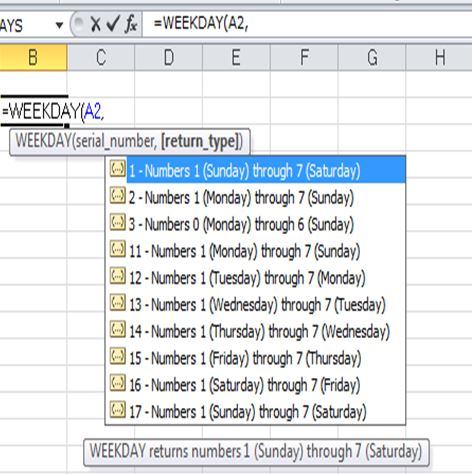NB: the Same date returns different day of the week numbers based on the return type. 1 is the default return type.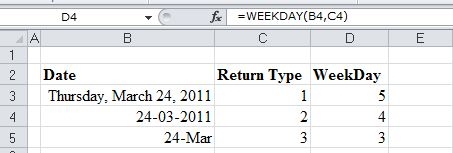# Create Summaries by Weekday or Weekend

When used together with SUMPRODUCT, WEEKDAY can be used to summarize data by days of the week.

For example, how do you know which is your best sales day?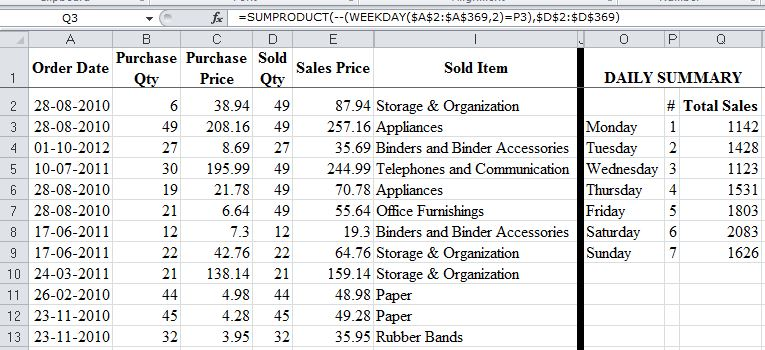From the  above, we can tell Saturday is our Best sales day by using  below formula

` =SUMPRODUCT(--(WEEKDAY(salesdate,2)=dayNumber)*Values)`

How it Works:

1. (WEEKDAY(salesdate,2)=dayNumber)→→generates an array of TRUE and FALSE  e.g. based on date number
` {FALSE,TRUE,FALSE,TRUE,FALSE,TRUE,FALSE....}`
1. This  Boolean array is converted to Numbers equivalent by use of double negatives (double unary Method).
`{0;1;0;1;0;1;0.....}`
1. SUMPRODUCT gets the sum of the product of this array of numbers and Qty Sold.
`=SUMPRODUCT({0;1;0;1;0;1;0....},{49,49,27,49,49,49,12....})`

# Count If by Day of the Week

Still based on above example, what if you wanted to know the number of transactions per day of the week.

You can use SUMPRODUCT and WEEKDAY again to count the number of day of week there are in all these sales transactions`=SUMPRODUCT(- -(WEEKDAY(salesdate,2)=dayNumber))`

How it Works:

As outlined above but now SUMPRODUCT just sums the array of 1 and 0 {0;1;0;1;0;1;0;1;1;0….}

# Create a Custom Day of the Week Name

With the help of another Overlooked function–CHOOSE, WEEKDAY can be used to create custom names.

For example return day of the week in Swahili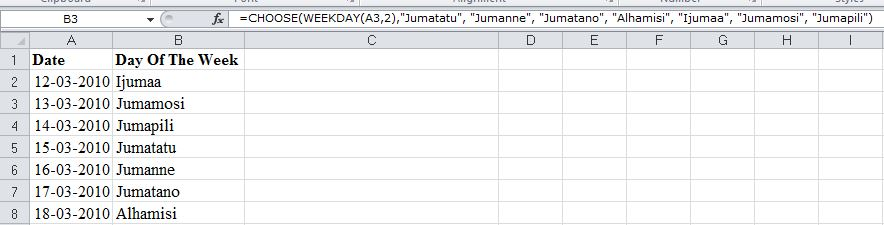`=CHOOSE(WEEKDAY(A3,2),"Jumatatu", "Jumanne", "Jumatano", "Alhamisi", "Ijumaa", "Jumamosi", "Jumapili")`

# Highlight Weekends or Weekdays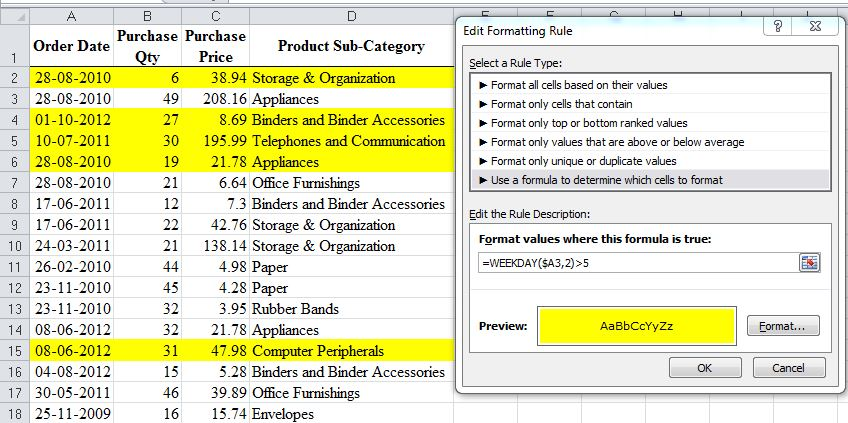Go to Conditional formatting→→New Rule→→Use Formula to Determine which Cell to Format

Apply this format  =WEEKDAY(firstCell with Date,2)>5→→This will format weekends

For formatting, Weekdays use this  =WEEKDAY(firstCell with Date,2)<=5

Finally, Select the area to apply the format# Count the number of specific weekdays/weekends between two dates with formulaThere are two ways of counting these days:

• using a combination of INT and WEEKDAY→→
` =INT((WEEKDAY(StartDate-DayofWeek)-StartDate + EndDate))/7)`
• Or combine,SUMPRODUCT,WEEKDAY,ROW & INDIRECT→→
` =SUMPRODUCT(--(WEEKDAY(ROW(INDIRECT(StartDate &":"& EndDate)))=DayofWeek))`

# Find the Nth Day of the Week of Any Month of Any Year

How can you Schedule meetings on every 4th Wednesday of Every month for the year 2017?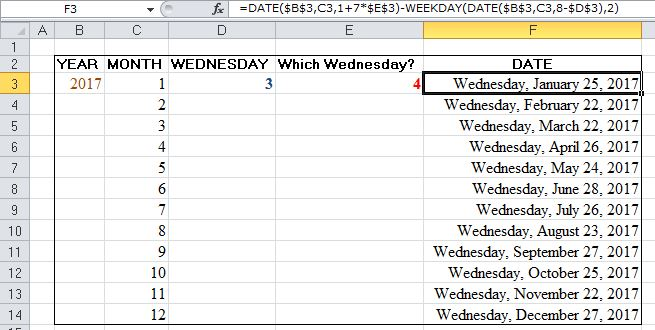` =DATE(year,month,1+7*NthDay)-WEEKDAY(DATE(year,month,8-DayofWeek),2)`

For example to calculate 4th Wednesday for May  =Date(2017,5,1+7*4)-WEEKDAY(DATE(2017,5,8-3),2)

### How it Works:

See this  link for full explanation

## RECOMMENDATION

7 OVERLOOKED USES OF EXCEL MOD FUNCTION

7 WAYS TO USE EXCEL REPT FUNCTION